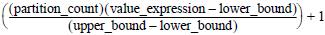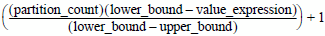# 17.00 - 17.05 - Rules - Advanced SQL Engine - Teradata Database

## Teradata Vantage™ - SQL Functions, Expressions, and Predicates

Product
Release Number
17.00
17.05
Published
June 2020
Language
English (United States)
Last Update
2021-01-30
dita:mapPath
tpt1555966086716.ditamap
dita:ditavalPath
lze1555437562152.ditaval
The following rules apply to WIDTH_BUCKET:
• If any argument is null, then the result is also null.
• If partition_count <=0 or if partition_count > 2147483646, an error is returned to the requestor.
• If lower_bound = upper_bound, an error is returned to the requestor.
• If lower_bound < upper_bound, then the rules in the following table apply.
IF … THEN the result is …
value_expression < lower_bound 0.
value_expression >= upper_bound partition_count +1.

If the result cannot be represented by the data type specified for the result, then an error is returned.

anything else the greatest exact numeric value with scale 0 that is less than or equal to the following expression.• If lower_bound > upper_bound, then the rules in the following table apply.
IF … THEN the result is …
value_expression > lower_bound 0.
value_expression <= upper_bound partition_count +1.

If the result cannot be represented by the data type specified for the result, then an error is returned.

anything else the least exact numeric value with scale 0 that is less than or equal to the following expression.